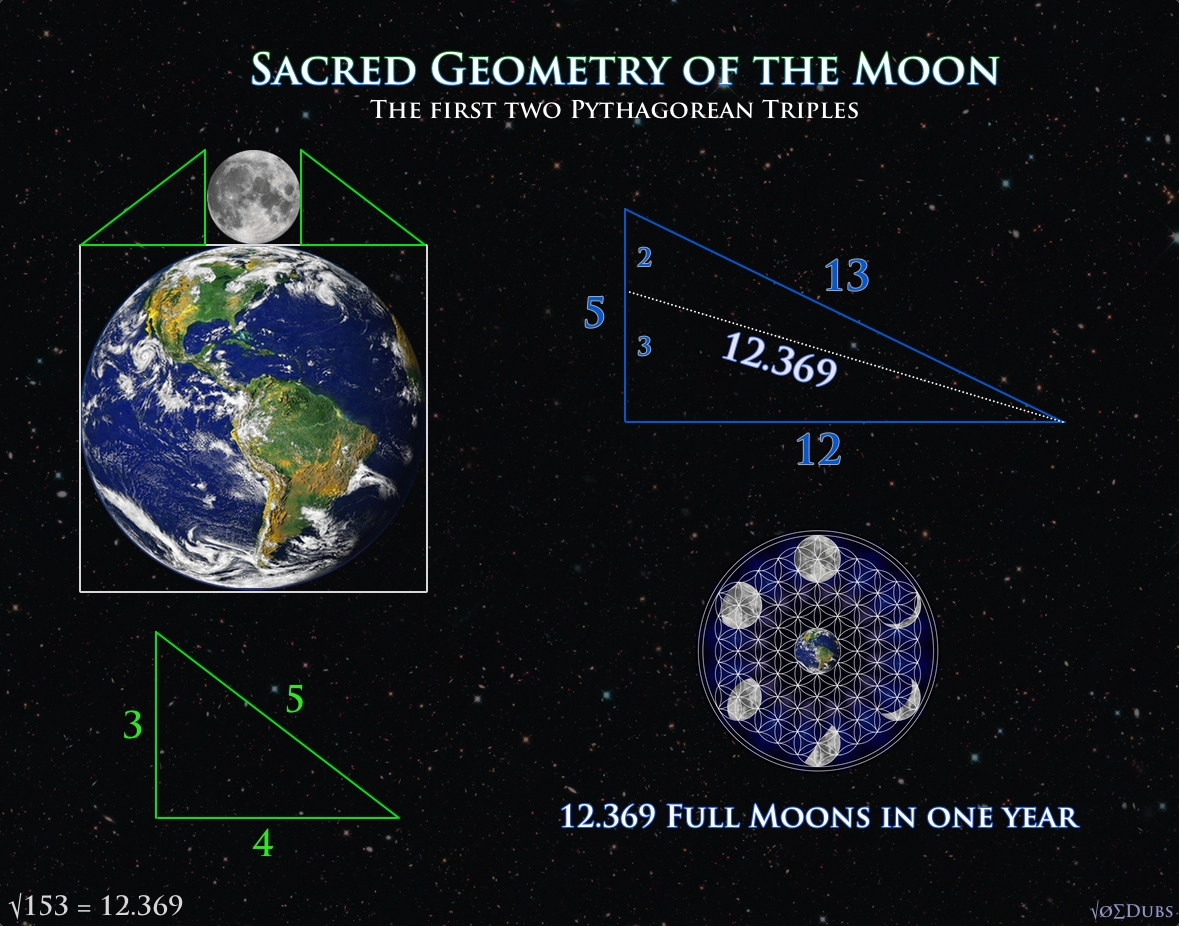## Sacred Geometry of the MoonThe physical dimensions of Earth and Moon reveal astounding geometries. The sacred geometry of the Moon was understood long ago and the ancients hid the esoteric meaning in the dimensions of their monuments and architecture. The Moon encodes the first two Pythagorean Triples, which are sets of whole numbers that satisfy the equation popularized by Pythagoras that everyone is familiar with. Pythagorean Theorem a^squared + b^squared = c^squared In the book “Taking Measure”, Scott Onstott shows that the point of intersection that defined the amount of full moons in a year (12.369), lies on the perfect fifth (2:3) ratio on the smallest side of the triangle. These numbers also encode esoteric knowledge about the geometric locations of Stonehenge and other sacred sites in England. He continues: “In The Lost Science of Measuring the Earth, John Michell and Robin Heath identified a 5:12:13 Pythagorean triangle…

3